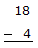# Digital Electronics - Digital Arithmetic Operations and Circuits

### Exercise :: Digital Arithmetic Operations and Circuits - General Questions

16.

The binary subtraction 0 – 0 =

 A. difference = 0borrow = 0 B. difference = 1borrow = 0 C. difference = 1borrow = 1 D. difference = 0borrow = 1

Answer: Option A

Explanation:

No answer description available for this question. Let us discuss.

17.

Convert each of the decimal numbers to 8-bit two's-complement form and then perform subtraction by taking the two's-complement and adding.A. 0001  0011 B. 0000  1110 C. 0010  1110 D. 1110  0000

Answer: Option B

Explanation:

No answer description available for this question. Let us discuss.

18.

Adding in binary, a decimal 26 + 27 will produce a sum of:

 A. 111010 B. 110110 C. 110101 D. 101011

Answer: Option C

Explanation:

No answer description available for this question. Let us discuss.

19.

Convert each of the following signed binary numbers (two's-complement) to a signed decimal number.
00000101        11111100        11111000

 A. –5    +4    +8 B. +5    –4    –8 C. –5    +252    +248 D. +5    –252    –248

Answer: Option B

Explanation:

No answer description available for this question. Let us discuss.

20.

How many basic binary subtraction operations are possible?

 A. 4 B. 3 C. 2 D. 1

Answer: Option A

Explanation:

No answer description available for this question. Let us discuss.

#### Current Affairs 2021

Interview Questions and Answers